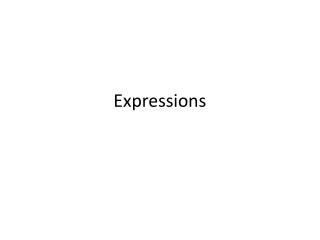DownloadDownload PresentationExpressions

# Expressions

Télécharger la présentation## Expressions

- - - - - - - - - - - - - - - - - - - - - - - - - - - E N D - - - - - - - - - - - - - - - - - - - - - - - - - - -
##### Presentation Transcript

1. Expressions

2. 2 x n is written as 2n • 1 x n or n x 1 is written as n • p x q or q x p is written as pq • (a + b) ÷ c is written as a + b c • X x (y + 4) is written as x(y + 4) • 3 x 2a is written as 6a • n x n is written as n2 and is said ‘n squared’ • n x n x n = n3 and is said ‘n cubed’ • n x n x n x n = n4 and said as ‘n to the power of 4’ • n x n x n x n x n = n5 and said as ‘n to the power of 5’ • 2n x 2n is written as (2n)2 or 4n2 • N x

3. Quiz • Write these without multiplication or division signs • 9 x (x + 3) • m x m • (n + m) ÷ p • 3a x 3a • 4p x p2 (squared)

4. Quiz • Write these with multiplication signs • 4p • 4(x + 3) • 5b2 (squared) • 8pq • (4n)2

5. Quiz • Write an expression for these • Three times the value of d • One third of n • Two times the product of m and n • Half the sum of a and b • Three times the sum of f and g

6. Quiz • Write true or false for these • 3 + n = n + 3 • 3b = 3 + b • (a+b) x 3 = 3(a+b) • ax b x 4 = 4ab • a – b – c + d = a + b + c - d